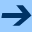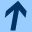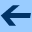The formal translation equation for iteration groups of type II

## The formal translation equation for iteration groups of type II

Jointly written with LUDWIG REICH.

Aequationes mathematicae 79, 111-156, 2010.

Abstract: We investigate the translation equation

(T) F(s+t,x)=F(s,F(t,x)),        s,t∈ ℂ,
in C[[x]], the ring of formal power series over ℂ. Here we restrict ourselves to iteration groups of type II, i.e. to solutions of (T) of the form F(s,x)≡ x+ck(s)xkmodxk+1, where k≥ 2 and ck ≠ 0 is necessarily an additive function. It is easy to prove that the coefficient functions cn(s) of
 F(s,x)=x+.. ∑n≥ k cn(s)xn..
are polynomials in ck(s).

It is possible to replace this additive function ck by an indeterminate. In this way we obtain a formal version of the translation equation in the ring (ℂ[y])[[x]]. We solve this equation in a completely algebraic way, by deriving formal differential equations or an Aczél-Jabotinsky type equation. This way it is possible to get the structure of the coefficients in great detail which are now polynomials. We prove the universal character (depending on certain parameters, the coefficients of the infinitesimal generator H of an iteration group of type II) of these polynomials. Rewriting the solutions G(y,x) of the formal translation equation in the form n≥ 0φn(x)yn as elements of (ℂ[[x]])[[y]], we obtain explicit formulas for φn in terms of the derivatives H(j)(x) of the generator H and also a representation of G(y,x) as a Lie-Gröbner series. Eventually, we deduce the canonical form (with respect to conjugation) of the infinitesimal generator H as xk+hx2k-1 and find expansions of the solutions G(y,x)=r≥ 0 Gr(y,x)hr of the above mentioned differential equations in powers of the parameter h.

harald.fripertinger "at" uni-graz.at, October 10, 2019